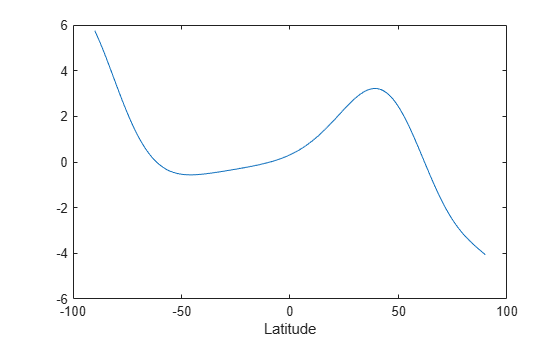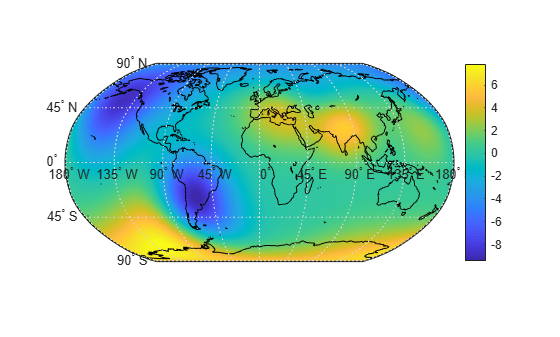# geopeaks

Generate synthetic data set on sphere

## Syntax

``Z = geopeaks(lat,lon)``
``Z = geopeaks(R)``
``Z = geopeaks(___,spheroid)``

## Description

example

````Z = geopeaks(lat,lon)` evaluates a "peaks-like" function at specific latitudes and longitudes on the surface of a sphere, returning the synthetic data set `Z`. The function is continuous and smooth at all points, including the poles. Reminiscent of the MATLAB `peaks` function, `geopeaks` undulates gently between values of `-10` and `8`, with about a half dozen local extrema.```

example

````Z = geopeaks(R)` evaluates the `geopeaks` function at cell centers or sample posting points defined by a geographic raster reference object, `R`.```

example

````Z = geopeaks(___,spheroid)` evaluates the function on a specific spheroid. The choice of spheroid makes very little difference; this option exists mainly to support formal testing.```

## Examples

collapse all

Define latitude and longitude values along meridian that includes Paris, France.

```lon = dms2degrees([2 21 3]); lat = -90:0.5:90;```

Generate a data set, specifying a spheroid.

`z = geopeaks(lat, lon, wgs84Ellipsoid);`

Display the profile.

```figure plot(lat,z) xlabel('Latitude')```Create a raster reference object for a 181-by-361 grid of postings.

```latlim = [-90 90]; lonlim = [-180 180]; sampleSpacing = 1; R = georefpostings(latlim,lonlim,sampleSpacing,sampleSpacing)```
```R = GeographicPostingsReference with properties: LatitudeLimits: [-90 90] LongitudeLimits: [-180 180] RasterSize: [181 361] RasterInterpretation: 'postings' ColumnsStartFrom: 'south' RowsStartFrom: 'west' SampleSpacingInLatitude: 1 SampleSpacingInLongitude: 1 RasterExtentInLatitude: 180 RasterExtentInLongitude: 360 XIntrinsicLimits: [1 361] YIntrinsicLimits: [1 181] CoordinateSystemType: 'geographic' AngleUnit: 'degree' ```

Generate a data set.

`Z = geopeaks(R);`

Display the resultant data set on a map.

```figure worldmap world geoshow(Z,R,'DisplayType','surface','CData',Z,'ZData',zeros(size(Z))) load coastlines geoshow(coastlat,coastlon,'Color','k') colorbar```## Input Arguments

collapse all

Geodetic latitude of one or more points, specified as a scalar value, vector, or matrix. Values must be in degrees.

The `lat` input argument must match the `lon` input argument in size unless either value is scalar (in which case it will expand in size to match the other), or `lat` is a column vector and `lon` is a row vector (they will expand to form a plaid latitude-longitude mesh).

Example: `lat = -90:0.5:90`

Data Types: `single` | `double`

Geodetic longitude of one or more points, specified as a scalar value, vector, or matrix. Values must be in degrees.

The `lon` input argument must match the `lat` input argument in size unless either value is scalar (in which case it will expand in size to match the other), or `lon` is a column vector and `lat` is a row vector (they will expand to form a plaid latitude-longitude mesh).

Example: `lon = -180:0.5:180`

Data Types: `single` | `double`

Geographic raster, specified as a `GeographicCellsReference` or `GeographicPostingsReference` object. The geographic raster stores the latitude and longitude of points.

Example: ```R = georefcells([0 80], [-140 60], 0.25, 0.25)```

Reference spheroid, specified as a `referenceEllipsoid`, `oblateSpheroid`, or `referenceSphere` object.

Example: `spheroid = referenceEllipsoid('GRS80')`

## Output Arguments

collapse all

Synthetic data set, returned as a scalar value, vector, or matrix of class `single` or `double`, depending on the class of the input. The function is evaluated at each element of `lat` and `lon` (following expansion as noted above), or at each cell center or posting point defined by `R`.